## How to use IF function with AND, OR, and NOT in Excel?

In Excel, the IF function is quite useful to check whether a cell meets a condition and return different values based on evaluated results (True and False). And combining the IF function and AND, OR, NOT functions, it will vary conditions to check cells. Here I will introduce how to use IF and AND functions, IF and OR functions, and combine multiple IF functions in Excel.

Supposing we have a sales table as the following screen shot shown, and now we want to evaluate the sale values and group them as "Unordinary", "Low", "Middle", and "High".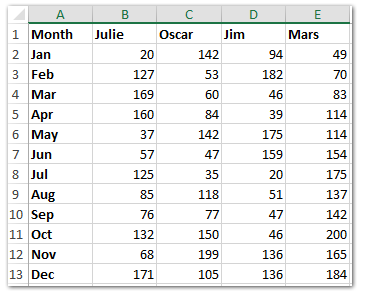And Now copy the row headers and column headers into a blank range (Range A16:E28 in our example) as the following screen shot shown.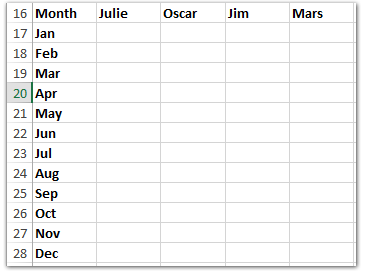#### How to use IF function in Excel

The basic form of IF function in Excel is shown as: =IF(logic_test, value_if true, value_if_false)

In our case we want to check the sale volumes are Low or not. If the value in Cell B2 is equal or less than 80, return the text of "Low", if the value in Cell B2 is bigger than 80, return blank.

Enter the formula =IF(B2<=80,"Low","") into the Cell B17, and then drag the Fill Handle to the Range B17:E28. See screen shot below: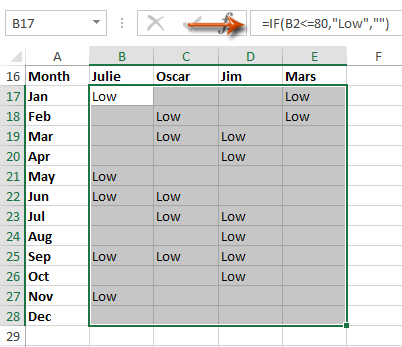#### Easily combine multiple worksheets/workbooks/CSV files into one worksheet/workbook

It may be tedious to combine dozens of sheets from different workbooks into one sheet. But with Kutools for Excel’s Combine (worksheets and workbooks) utility, you can get it done with just several clicks!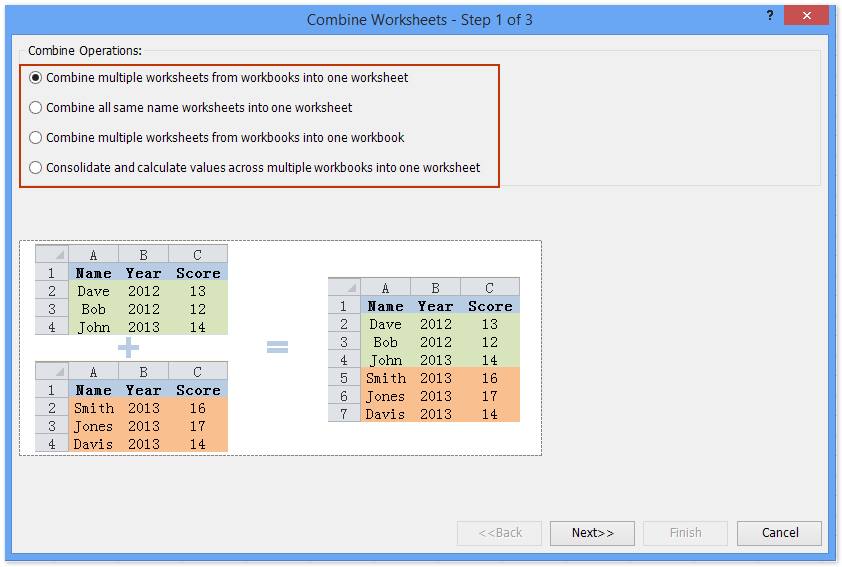#### How to use If function and AND function in Excel

If you want to check if a cell meets several conditions at the same time, you can combine the IF function and AND function in Excel.
The basic form of AND function in Excel is =AND(Logical 1, Logical 2, Logical 3, …, etc.)

Now in our case we want to check a sale volume is more than 80 and less than 160 simultaneously, if yes returns the text of "Middle", and if no returns blank.

Enter the formula =IF(AND(B2>80,B2<160),"Middle","") into Cell B17, and then drag the Fill Handle to the Range B17:E28. See screen shot below: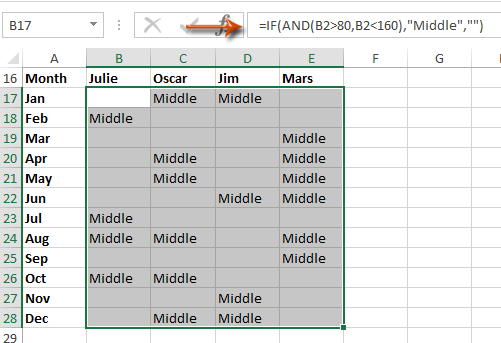#### How to use IF function and OR function in Excel

If you want to check if a cell meets one of multiple conditions, you can combine the IF function and OR function in Excel.

The basic form of OR function in Excel is =OR(Logical 1, Logical 2, Logical 3, …, etc.)

In our example, we want to find out the unordinary sale volumes which are bigger than 180 or less than 20. If the sale volumes are unordinary, return the text of "Unordinary", if no return blank.

Enter the formula =IF(OR(B2>180,B2<20),"Unordinary","") into Cell B17, and then drag the Fill Handle to the Range B17:E28. See screen shot below:#### How to combine multiple IF functions into one formula in Excel

In our example, if you want to evaluate all sale volumes with one formula, you can apply a complex IF function.

If the sale volume is equal or less than 20, return text of "Unordinary"; if the sale volume is equal or less than 80, return text of "Low"; if equal or less than 160, return text of "Middle", and if equal or less than 200, return text of "High".

Enter the formula =IF(B2<=20,"Unordinary",IF(B2<=80, "Low", IF(B2<=160, "Middle", IF(B2<=200, "High", "")))) into Cell B17, and then drag the Fill Handle to the Range B17:E28. See screen shot below: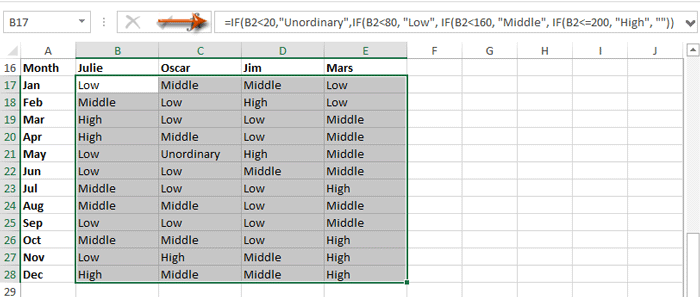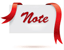Formula is too complicated to remember? Save the formula as an Auto Text entry for reusing with only one click in future! Read more…     Free trial

### Best Office Productivity Tools

#### Supercharge Your Spreadsheets：Experience Efficiency Like Never Before with Kutools for Excel

 Popular Features: Find/Highlight/Identify Duplicates   |  Delete Blank Rows   |  Combine Columns or Cells without Losing Data   |   Round without Formula ... Super Lookup: Multiple Criteria VLookup  |   Multiple Value VLookup  |   VLookup Across Multiple Sheets   |   Fuzzy Lookup .... Advanced Drop-down List: Quickly Create Drop Down List   |  Dependent Drop Down List   |  Multi-select Drop Down List .... Column Manager: Add a Specific Number of Columns   |   Move Columns   |   Unhide Columns   |   Compare Columns to Select Same & Different Cells ... Featured Features: Grid Focus   |  Design View   |   Big Formula Bar   |  Workbook & Sheet Manager   |  Resource Library (Auto Text)   |  Date Picker   |  Combine Worksheets   |  Encrypt/Decrypt Cells   |  Send Emails by List   |  Super Filter   |   Special Filter (filter bold/italic/strikethrough...) ... Top 15 Toolset:  12 Text Tools (Add Text, Remove Characters, ...)   |   50+ Chart Types (Gantt Chart, ...)   |   40+ Practical Formulas (Calculate age based on birthday, ...)   |   19 Insertion Tools (Insert QR Code, Insert Picture from Path, ...)   |   12 Conversion Tools (Numbers to Words, Currency Conversion, ...)   |   7 Merge & Split Tools (Advanced Combine Rows, Split Cells, ...)   |   Many More...

Kutools for Excel boasts over 300 features, ensuring that what you need is just a click away...

Supports Office/Excel 2007-2021 & newer, including 365   |   Available in 44 languages   |   Enjoy a full-featured 30-day free trial.#### Office Tab Brings Tabbed interface to Office, and Make Your Work Much Easier

• Enable tabbed editing and reading in Word, Excel, PowerPoint, Publisher, Access, Visio and Project.
• Open and create multiple documents in new tabs of the same window, rather than in new windows.
• Increases your productivity by 50%, and reduces hundreds of mouse clicks for you every day!No ratings yet. Be the first to rate!
This comment was minimized by the moderator on the site
Hey Friends,

Donno if this Forum works now:

I am trying to figure out a formula for the following:
I have 3 Columns : viz : A1,D1, L1 with text contents. And output expected in M1

So if any of the cells A,D,L has Faulty mentioned, then M1 should result "Faulty", Else if all 3 are blank , need "Spare", and if any cell has any data then it shoud show "Mapped"
This comment was minimized by the moderator on the site
Kan iemand mij helpen met onderstaande voor het maken van een formule?
als B2 de tekst factuur staat moet in cel D8 de tekst factuurdatum komen te staan. Als in cel B2 de tekst offerte staat moet in cel D8 offertedatum komen te staan.

Alvast hartelijk dank
This comment was minimized by the moderator on the site
Hi there,

Can you show us the look of the text invoice, text quotation and text quotation date?
This comment was minimized by the moderator on the site
I am trying to write a formula to populate scores based off a range of values. This is what I have so far:

=IF(C2>=104.5%,"5", IF(C2<=104.49%,"4", IF(C2>=95.5%,"4", IF(C2<=95.49%,"3", IF(C2>=79.5%,"3", IF(C2<=79.49%,"2", IF(C2>=59.5%,"2", IF(C2<=59.49%,"1"""))))))))

The formula is working and I am not receiving any error messages. However, it is not populating the lower range values correctly. Here is my range:

>105% = 5
96%-105% = 4
80%-95% = 3
60%-79% = 2
<60% = 1

Any help is greatly appreciated.
This comment was minimized by the moderator on the site
Hi there,

I've fixed the formula as shown below:
=IF(C2>105%,"5",IF(C2>=96%,"4",IF(C2>=80%,"3",IF(C2>=60%,"2","1"))))

Hope this is what you want.

Amanda
This comment was minimized by the moderator on the site
Never mind, I figured it out. Thanks!
This comment was minimized by the moderator on the site
Kan iemand mij helpen aan onderstaande formule?
Alvast bedankt!

als Q groter is dan C dan Q en als Q kleiner is dan H dan H tenzij H 0 is dan is Q
This comment was minimized by the moderator on the site
Hi there,

Suppose, Q=A1, C=A2, H=A3. Please use the formula below: =IF(B1>B2,B1,IF(B1<B3,IF(B3=0,B1,B3),B3))

Hope this is what you want.

Amanda
This comment was minimized by the moderator on the site
I am trying to find a formula when realignment is in column A then add the text conflict when transition begins date (B) is >= the solution start date in column D OR if activation in column A then add the text conflict when transition begins date is <= solution start date in column D OR if deactivation then add the text conflict when transition begins date is >= the solution start date
A B C D E
Transition Type Transition Begins Transition Ends Solution Start Date Solution End Date
Realignment 11/1/2022 11/15/2022 1/15/2022 3/6/2022
Realignment 11/1/2022 11/15/2022 1/15/2022 3/16/2022
Realignment 11/1/2022 11/15/2022 6/1/2022 7/16/2022
Realignment 11/1/2022 11/15/2022 6/1/2022 7/16/2022
Realignment 11/1/2022 11/15/2022 6/1/2022 7/16/2022
Activation 1/1/2022 1/31/2022 1/15/2022 3/6/2022
Deactivation 12/1/2021 12/15/2021 1/15/2022 3/6/2022
Reorganization 2/6/2022 2/12/2022 1/15/2022 3/6/2022
Activation 12/1/2021 12/31/2021 11/1/2029 12/31/2029
Activation 12/1/2021 12/31/2021 2/1/2025 7/31/2025
Activation 12/1/2021 12/31/2021 4/1/2024 6/29/2024
Activation 12/1/2021 12/31/2021 2/1/2028 3/2/2028
Activation 12/1/2021 12/31/2021 2/1/2022 5/20/2025
Activation 12/1/2021 12/31/2021 9/6/2022 3/16/2023
Activation 12/1/2021 12/31/2021 6/1/2024 11/28/2024
Activation 12/1/2021 12/31/2021 9/1/2022 9/7/2022
Deactivation 10/1/2021 10/30/2021 11/1/2029 12/31/2029
Deactivation 10/1/2021 10/30/2021 2/1/2025 7/31/2025
Deactivation 10/1/2021 10/30/2021 4/1/2024 6/29/2024
Deactivation 10/1/2021 10/30/2021 2/1/2028 3/2/2028
Deactivation 10/1/2021 10/30/2021 2/1/2022 5/20/2025
Deactivation 10/1/2021 10/30/2021 9/6/2022 3/16/2023
Deactivation 10/1/2021 10/30/2021 6/1/2024 11/28/2024
Deactivation 10/1/2021 10/30/2021 9/1/2022 9/7/2022
Reorganization 2/1/2022 2/28/2022 11/1/2029 12/31/2029
Reorganization 2/1/2022 2/28/2022 2/1/2025 7/31/2025
This comment was minimized by the moderator on the site
Hi there,

What do you mean by adding the text conflict? Can you show me the result you want?

Amanda
This comment was minimized by the moderator on the site
=IF(AND(AY7>60,AY7>30),"0.02","0.04"),if(and(ay7<=30,ay<az),"0.06"),if(and(ay7<=15,ay<az),"0.08")
This comment was minimized by the moderator on the site
I am trying use If formula for one oridinary file, lets say I have number from 1 to 31 in a perticular cell. I need to show the result in another cell as if number in that perticular cell is less than 26 they it will zero, if the number in that perticular cell is from 26 to 30 then it will show the same number but if the number is abobe 30 then it will show the 30 only....Can anyone advise me how can I formulate this formula?
This comment was minimized by the moderator on the site
Hi there,

Please try the formula below: =IF(A1<26,0,IF(A1<=30,A1,30))

Amanda
This comment was minimized by the moderator on the site
Ciao,
mi potete aiutare perfavore...
ho tre celle:
1-data di pagamento
2-totale
3-totale se pagato

vorrei che quando inserisco la data di pagamento(1), la casella 3(che è vuota) si riempisse automaticamente come la casella 2
come posso fare?
This comment was minimized by the moderator on the site
Hi there,

Do you want to fill the value of the cell 2 in the cell 3?
If so, you can enter this IF formula in the casella 3: =IF(casella 1<>"",casella 2,"")

Amanda
This comment was minimized by the moderator on the site
=IF(D4<=30000, and =>20000,than D4a-5000,and if(d4<=40000, and >30000, than d4-6000) convert into formula
This comment was minimized by the moderator on the site
Try the formula. Since I don't quite understand you, please check and change the part "DA4-5000" and "D4-6000", "FALSE" to the results you want.
=IF(AND(D4<=30000,D4>20000),"DA4-5000",IF(AND(D4<=40000,D4>30000),"D4-6000","FALSE"))
Amanda
This comment was minimized by the moderator on the site
HI EVERY ONE I NEED HELP IN THIS SYNTEX=IF(AND(AJ=1250,AJ*2.5%),IF(AND(AJ>1250,AJ<=2500),AJ*10%,IF(AND(AJ>2500,AJ<=3750),AJ*15%,IF(AND(AJ>3750,AJ<=11666),AJ*20%))))
THIS FOURMAIL GIVE ME #NAME WHERE THE EROO
This comment was minimized by the moderator on the site
Hi ahmed.dba,
Can you send the file to ? And if you have private information in the file, please delete them.
Amanda
This comment was minimized by the moderator on the site
I am trying to write a formula to tell me if I am available or busy on specific days and dates (I am always busy on certain days of the week, but then also on specific dates).What I have so far is:=IF(OR(A226="Monday",A226="Wednesday",A226="Thursday",A226="Friday",\$Z\$226:\$Z\$255=B226),"Busy","Available")
Columns A is the day of the week as text, column B is the day of the month as a number,
This comment was minimized by the moderator on the site
Hi Andrea,

Can you send the file to ? And if you have private information in the file, please delete them.

Amanda
This comment was minimized by the moderator on the site
I need help to make a formula Quantity Count 1 Count 2 Count 3
10837 10874 10874 0
If count =0, make count 2-Quantity, if is <> 0, make count 3-Quantity, and if count2 and count 3 =0, make count 1-Quantity
This comment was minimized by the moderator on the site
I need help combining these IF formula:
IF D7<>C\$1 "OOP", "Current"
IF D7=C\$1 and S7=0, T7>0, "No Rev"
IF D7=C\$1 and S7<0, T7> 0, "Loss"
IF D7=C\$1 and S7<0, R=0, "No Cost"
IF D7=C\$1 and X7<=30%, "High GP"
IF D7-C\$1 and X7>=5%, "Low GP"
This comment was minimized by the moderator on the site

=IF fr2=31 (FT4>30,18000,FR4*FQ4) and IF fr2=30 (FT4>29,18000,FR4*FQ4)

i want to combine this.

This comment was minimized by the moderator on the site
Hi there, I need this to return the value in the column B6:B28, when either an H or an S are in the boxes I6:I28, works fine without the OR and just H, but not with the OR function, can you possibly help?=IF(AF8=I5,TEXTJOIN(",",FALSE,IF(OR(I6:I28="H",I6:I28="S"),B6:B28,"")))

Many thanks

David
This comment was minimized by the moderator on the site

If A1 begins with 2 zeros (00) return the number 1, if not return the number 2
This comment was minimized by the moderator on the site
8. To be a Bunk Leader, a staff member must have over 4 service years and have completed leadership training.
In cell L2, enter a formula using the IF and AND functions, as well as structured references to determine if Adam Moriarty can be a bunk leader.
a. The logical test in the IF function should determine if the staff member’s Service Years is greater than 4 AND the staff member’s Leadership Training status is “Yes”. Remember to use a structured reference to the Service Years and the Leadership Training columns.
b. The function should return the text Yes if a staff member meets both of those criteria.
c. The function should return the text No if a staff member meets none or only one of those criteria.
This comment was minimized by the moderator on the site
Hi Alice,
Supposing the number of service year is placed in Cell B2, the leadership training stats is placed in Cell C2, you can use this formula =IF(AND(B2>4,C2="Yes"),"Yes","No")
This comment was minimized by the moderator on the site
hello!
I need your brilliant minds to resolve a problem:
if a1=blank & b1=blank & c1=blank & d1=blank then result1
if a1=blank & b1=blank & c1=blank & d1>0 then result2
if a1=blank & b1=blank & c1>0 & d1>0 then result3
if a1=blank & b1>0 & c1>0 & d1>0 then result4
if a1>0 & b1>0 & c1>0 & d1>0 then result5
i've tried with if/and, if/or but it seams i can't get a result.

This comment was minimized by the moderator on the site
``=IF(AND(ISBLANK(A1);ISBLANK(B1);ISBLANK(C1);ISBLANK(D1));1;IF(AND(ISBLANK(A1);ISBLANK(B1);ISBLANK(C1);D1>0);2;IF(AND(ISBLANK(A1);ISBLANK(B1);C1>0;D1>0);3;IF(AND(ISBLANK(A1);B1>0;C1>0;D1>0);4;5))))``
This comment was minimized by the moderator on the site
Need some help with logic. I wanted to check the first cell for data if it has data then it will return that cell; if it doesn't have data then it will return a different cell. But if the second cell is blank then it should return a msg. Can you please help.
This comment was minimized by the moderator on the site
Hi Ahmed,
Supposing the first data in Cell A1, and the second data in Cell A2, you can apply the formula as =IF(A1="",IF(A2="","NO DATA", A2),A1)
This comment was minimized by the moderator on the site
IF(D15>30,"Organic Soils",IF(AND(D15>25,D15<29.99),"Clayey Soil (fine)",IF(AND(D15>20,D15<24.99),"Loamy Soil (moderately fine)",IF(AND(D15>10,D15<19.99),"Loamy Soil (medium)",IF(AND(D15>5,D15<9.99),"Loamy Soil (moderately coarse)",IF(D15<4.99,"Sandy Soil (coarse)")))))) Can you find the error?
This comment was minimized by the moderator on the site
A,b,c,d,e,f,g,a,y
This comment was minimized by the moderator on the site
Name Amount Profit Range
A 10,000
B 50,000
C 42,500
D 75,000
E 85,000
F 23,500
G 16,000
I 57,000
J 81,000

Profit Range Name
Less than 10,000 A
10,000-20,000 B
20,000-30,000 C
30,0000-50,000 D
50,0000-75,000 E
75,000-1,00,000 F

Write profit range in 1st table.
any Please say how to write formula for this
This comment was minimized by the moderator on the site
i required how to steel calculation as per dia (8,10,12,16,20,25,32) and cutting length no of bars and numbers etc

wise in excel format
This comment was minimized by the moderator on the site
how to use if condition for condition like if value of x lying in 60-80
This comment was minimized by the moderator on the site
Hello all,

I am trying to build an IF statement to help with our Service Level calculations. We need one Queue Name to have a different time requirement than all of the other queues. I am trying to figure out how to get the formula to count all Queue Names (Column B) to show they are within our Service Level Agreement (SLA) if they are in the queue for less than 30 seconds. However, for the Queue named TS_DX_CP_ES*, I need it to show it is within SLA if less than 90 seconds.

I currently have the following formula in place and it works for the 90 second rule but if I try to next the IF formula and change the Queue Name and Time Requirement, I get an error.

Formula that works currently =IF(AND([@[Queue Name]]="TS_DX_CP_ES*",[@[Queue time]]<0.00104166666666667),1,0)

Formula that gives a #VALUE! Error - =IF(AND([@[Queue Name]]="TS_DX_CP_ES*",[@[Queue time]]<0.00104166666666667),1,0),IF(AND([@[Queue Name]]="TS_PLU_US*",[@[Queue time]]<0.000358796296296296),1,0)
This comment was minimized by the moderator on the site
Hello all,

I am trying to build an IF statement to help with our Service Level calculations. We need one Queue Name to have a different time requirement than all of the other queues. I am trying to figure out how to get the formula to count all Queue Names (Column B) to show they are within our Service Level Agreement (SLA) if they are in the queue for less than 30 seconds. However, for the Queue named TS_DX_CP_ES*, I need it to show it is within SLA if less than 90 seconds.

I currently have the following formula in place and it works for the 90 second rule but if I try to next the IF formula and change the Queue Name and Time Requirement, I get an error.

Formula that works currently =IF(AND([@[Queue Name]]="TS_DX_CP_ES*",[@[Queue time]]<0.00104166666666667),1,0)

Formula that gives a #VALUE! Error - =IF(AND([@[Queue Name]]="TS_DX_CP_ES*",[@[Queue time]]<0.00104166666666667),1,0),IF(AND([@[Queue Name]]="TS_PLU_US*",[@[Queue time]]<0.000358796296296296),1,0)

This comment was minimized by the moderator on the site
Hi,

In a row, 3 columns with different value must show a different interpretation in the target cell.

=IF(A3>0,"Welcome",IF(B3>0,"Win",IF(C3>0,"Will","HI")))

Thanks Pinaki
This comment was minimized by the moderator on the site
Hi Pinaki,
In your formula, If A3 > 0, both B3 and C3 also might be greater than 0. Similarly, when B3 > 0, both A3 and C3 also might be greater than 0.

Normally, in the If function, you can set =IF(A3>0, "Welcome",””), which means when A3>0, returns “welcome”, while when A3 is not less than 0, returns nothing. In this function, the two condition A3>0 and A3<=0 are exclusive.

In my example =IF(B2<=20,"Unordinary",IF(B2<=80, "Low", IF(B2<=160, "Middle", IF(B2<=200, "High", "")))), The third condition B3<=160 includes the second condition B2<=80, and the second contains the first too.
This comment was minimized by the moderator on the site
Column A has Numerical Values. Column C & D have text.

If 2 or more separate Numerical Values in any row of Column A match then evaluate those Rows in Column C & D for any Text that doesn't match a given Text and count as 1. If Text does match then a value of 0 is assigned.

So if..

A1:A2 = 1

A3:A4 = 2

C1=Dog
C2=Cat
C3=Dog
C4: Horse

D1=Cat
D2=Dog
D3=Horse
D4=Dog

Since A1 & A2 match each other, evaluate C1,C2,D1,D2 and if any cells do not match either "Dog" or "Cat" assign a value of 1. In this example, since all cells contain either "Dog" or "Cat" a value of 0 would be assigned.

Furthermore,

Since A3 & A4 match each other, evaluate C3,C4,D3,D4 and if any cells do not match either "Dog" or "Cat" assign a value of 1. In this example, since cells C4 & D3 contain something other than "Dog" or "Cat" a value of 1 would be assigned.

This comment was minimized by the moderator on the site
Hi Cab,
To know more about the IF function, I guess this webpage is helpful.
https://support.office.com/en-us/article/IF-function-69aed7c9-4e8a-4755-a9bc-aa8bbff73be2
This comment was minimized by the moderator on the site
Hello,
What would the equation for the following be?

In cell K2 enter a formula using the IF and OR functions, as well as structured references, to determine if Adam Moriarty can be a group leader.
a. The IF function should first determine if the staff member’s Service Years is greater than 3 OR if the staff member’s college graduate status is “Yes”. Remember to use a structured reference to the Service Years and the College Graduate columns.
b. The function should return the text Yes if a staff member meets one or both of those criteria.
c. The function should return the text No if a staff member meets neither of those criteria.
This comment was minimized by the moderator on the site
Hi jessjess,
Thank you for your comment. I am sorry I can’t figure out a proper formula to meeting your conditions. To know more about the IF function, I guess this webpage is helpful.
https://support.office.com/en-us/article/IF-function-69aed7c9-4e8a-4755-a9bc-aa8bbff73be2
This comment was minimized by the moderator on the site
This comment was minimized by the moderator on the site
Hi Haris,
This comment was minimized by the moderator on the site
III
II
II
II

II
II
II
II

How can I make a formula in excel -If any one value of the column is III the result shall be displayed as III, if any value of the column is II then the result shall be displayed as II if II and III not present it shall be displayed as I
This comment was minimized by the moderator on the site
How would I write this into one function?

If D26 and E26 are blank, then C26-L7
If D26 is not blank and E26 is, then D26-L7
If E26 is not blank, then E26-L7
This comment was minimized by the moderator on the site
=IF(AND(D26="",E26=""),(C26-L7),IF(AND(D26<>"",E26=""),D26-L7,E26-L7))
This comment was minimized by the moderator on the site
I am trying to write a formula to look at

1. Is the figure either over £1000, or under -£1000

2. Plus is the next cell over 55% or more

so far I have =OR(M4>=1000,M4<=-1000) which pulls in the over or under £1000 plus or minus but am unsure how to add the second criteria presume its an AND(N2>=55%) but when I add it to the formula it doesnt work.
This comment was minimized by the moderator on the site
Hi Michelle,
This comment was minimized by the moderator on the site
Hi. How can I write this as formula?

If year is equal to current year, add 1.
if year is previous year, add 1 OR start 1.
if year is 2 years prior, add 1 OR start 1.
This comment was minimized by the moderator on the site
Hi Everyone, I need to write a formula for the following:

IF A1 = "BOTTOMS" AND A9 <14, then 14, otherwise A9

OR A1 = "TOPS" AND A9 <5, then 5, otherwise A9
This comment was minimized by the moderator on the site
Hi FG，
You can’t create a formula to meeting one of both your conditions in Excel.
This comment was minimized by the moderator on the site
Hi Kelly!

I actually figured out a workaround!

``=ROUND((IFERROR((IF(OR(AND(\$I9="BOTTOMS",BL9>=\$BY\$3),AND(\$I9="TOPS",BL9>=\$BY\$4)),BL9,VLOOKUP(\$I9,\$BX\$3:\$BY\$4,2,FALSE))),0)),0)``

I9 - populates "BOTTOMS" or "TOPS" dependant on merchandise code entered
BL9 - minimum set defined by store indice
BY9 - minimum set defined by buyer (i.e the 14 units for bottom and and the 5 units for tops)
BX\$3:\$BY\$4 - vlookup to minimum set defined by buyer if if statement is false
This comment was minimized by the moderator on the site
Hello! I am currently creating a template that will provide me a value depending on the score of the trainee.

I was hoping I can add a value of 5 for any team member who gets a certain quiz %

Here's what I'm thinking.

5= 100%
4= 99.9% to 90%
3= 90% to 85%
2= 85% to 80%
1= <79.99

I hope you guys can help me with the formula
This comment was minimized by the moderator on the site
Hello, I have a pretty complex formula I am trying to write and it is killing me to admit defeat on this one. So far I have:

=IF(AND(B2="Prudential",OR(C2={"10 Year Term","15 Year Term","20 Year Term","30 Year Term"})),D2*0.75,(IF(C2="UL",D2*0.8,"0")))

and it works just fine. However, I need to add about 37 more companies with their own commission structures to it. I am pretty sure I just need one monster OR statement with a bunch of nested IFs thrown in I just am at my wits end on figuring out exactly where to place them. Any help would be amazing. Thank you!
This comment was minimized by the moderator on the site
Hi Dan,
Your question is still unclear. you might to explain more by providing a solution for at least 2 companies. However, just help to shorten your OR function a bit. OR(C2={"10","15","20","30"}&" Year Term").
This comment was minimized by the moderator on the site
=IF(OR((I2=(T2+U2),(I2=(U2+V2)),"Closed","OPEN")
HOW TO MAKE THIS RIGHT?
This comment was minimized by the moderator on the site
=IF(OR(I2=T2+U2,I2=U2+V2),"CLOSED","OPEN")
This comment was minimized by the moderator on the site
Im trying to do IF(OR(A1=1;AND(A2=1;A3=1)))
This comment was minimized by the moderator on the site
Hi Mirko,
Thank you for your comment. What calculation result do you want to get? Could you tell me more about your problem?
This comment was minimized by the moderator on the site
I'm trying to make the formula return 4 possible answer which is (R-regular,A-absent,L-late,OT-overtime). My basis for regular is if the value of the cell is "8". So this is the formula that I made so far =IF(H16>7.9,"OT",IF(H16<7.9,"L",IF(H16=8,"R","A"))). It only returns (OT and L) something is very wrong and i can't figure it out. I'm just new to excel so please help me
This comment was minimized by the moderator on the site
Hi Dan,
Try this formula =IF(H16=8,"R",IF(H16<7.9,"OT","L"))
This comment was minimized by the moderator on the site
I'm trying to make the formula return 4 possible answer which is (R-regular,A-absent,L-late,OT-overtime). My basis for regular is if the value of the cell is "8". So this is the formula that I made so far =IF(H16>7.9,"OT",IF(H16<7.9,"L",IF(H16=8,"R","A"))). It only returns (OT and L) something is very wrong and i can't figure it out. I'm just new to excel so please help me.
This comment was minimized by the moderator on the site
Try this: =IF(OR(H16=0,H16=""),"A",IF(H16=8,"R",IF(H16<7.9,"L","OT")))
This comment was minimized by the moderator on the site
how to determine the age of the cell ? is this formula right =IF(I2<30,"future payment",AND(IF(I2>30<=45,"30-45days",AND(IF(I2>45<=60,"45-60days",AND(IF(I2>60,"61+days
",0)))))))
This comment was minimized by the moderator on the site
Hi pramod,
You cannot use I2>30<=45 in formula directly. I have changed the formula for you: =IF(I2<=30,"FUTURE PAYMENT",IF(B2<=45, "30-45 DAYS", IF(B2<=45, "45-60DAYS", IF(B2<=60, "60+DAYS", ""))))
This comment was minimized by the moderator on the site
I have charges calculation for eg. charges amount is 0 then next column should be 0. if charges is more than 1 and less than 250 then next column should be 250. If charges is more than 250 then actual charges should come in next column(252)
This comment was minimized by the moderator on the site
then what about charge 1 ?

charge 0 = 0
Charge more than 1 but 250 = 250
Charge more than 250 = charge the actual value

"I have charges calculation for eg. charges amount is 0 then next column should be 0.
if charges is more than 1 and less than 250 then next column should be 250. If charges is more than 250 then actual charges should come in next column(252)"

Charge
0 0
45 250
250 250
255 255
300 300
0 0
1 FALSE
This comment was minimized by the moderator on the site
how do i make this formulas talk to one another: =IF(OR(AND(MID(K2,6,1)="N",(MID(K2,6,1)="C"),(MID(K2,6,1)="H"),(MID(K2,6,1)="I"),(MID(K2,6,1)="B"),(MID(K2,6,1)="F"),(MID(K2,6,1)="L"),(MID(K2,6,1)="M"),(MID(K2,6,1)="P"),(MID(K2,6,1)="R"),(MID(K2,6,1)="P"),ISTEXT(G2)="61"),OR(AND(RIGHT(K2,2)=G2))),"Good","Review") =IF(AND(RIGHT(K2,2)=G2),"Good","Review") or =IF(NOT(OR(AND(MID(K2,6,1)="N",(MID(K2,6,1)="C"),(MID(K2,6,1)="H"),(MID(K2,6,1)="I"),(MID(K2,6,1)="B"),(MID(K2,6,1)="F"),(MID(K2,6,1)="L"),(MID(K2,6,1)="M"),(MID(K2,6,1)="P"),(MID(K2,6,1)="R"),(MID(K2,6,1)="P"),ISTEXT(G2)="61"),(RIGHT(K2,2)=G2))),"Good","Review")
There are no comments posted here yet